# AP Physics C Electricity : Resistors

## Example Questions

### Example Question #1 : Electric Circuits

Resistors are one of the most important basic components of a circuit. With very few exceptions, all circuits have at least one kind of resistor component. An ammeter is a device that measures current flowing through a circuit. Ammeters are always connected to a circuit in series.

Which of the following accurately explains why ammeters must be connected in series within a circuit, and never in parallel?

An ammeter connected in parallel would give very low readings of current because current would be flowing through both the regular current path and the ammeter, but disproportionately through the regular path

An ammeter connected in parallel would not read any current because the current would have no driving force through the ammeter

An ammeter connected in parallel would give the current flowing through the circuit a pathway with minimal resistance, creating a very large current that could harm the ammeter

An ammeter connected in parallel would give readings of half the current flowing through the circuit because current would flow evenly between the ammeter and the circuit's regular pathway

An ammeter connected in parallel would give high readings of current because the current would be flowing through both the regular current path and the ammeter, but disproportionately through the ammeter

An ammeter connected in parallel would give the current flowing through the circuit a pathway with minimal resistance, creating a very large current that could harm the ammeter

Explanation:

In order to give accurate readings of current, ammeters have very low resistances. If connected in parallel, the voltage pushing current through the circuit would push a very strong current through the ammeter and virtually no current through the circuit's regular path. This would not only lead to a bad reading of current, but more often than not a broken ammeter. This phenomenon is an indication of why resistors are so important: they limit the current such that a circuit does not exceed its current-carrying capacity.

### Example Question #11 : Electric Circuits

A battery is measured to have a potential of 5V. When connected to a wire with no resistors or other components, the voltage measured is 4.9V.

Why was the potential of the battery measured differently when the wire was connected?

The wire connected to the battery must be connected to something else where this potential is being lost

The wire has a slight internal resistance and caused a potential drop

Once the current is distributed across the wire, there is less charge per unit length of the circuit and so the voltage is lower

The wire is causing electrons to be lost to the air, which lowers the potential measured

The difference in potential measured is small enough to be disregarded

The wire has a slight internal resistance and caused a potential drop

Explanation:

All wires have at least some internal resistance. The most likely explanation for this is that the wire is displaying slight resistance, and therefore caused the measured potential to be less than it was before.

### Example Question #11 : Electric Circuits

Three resistors and a battery form the following circuit.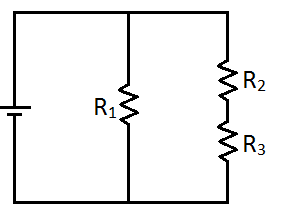Calculate the equivalent resistance if the resistors have the following values.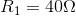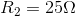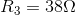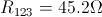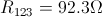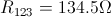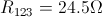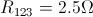Explanation:

First, calculate the equivalent resistance of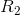and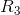. Since these two resistors are arranged in series, we just take the sum of their values.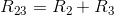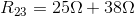With resistors 2 and 3 combined together in a single value, the following circuit is formed.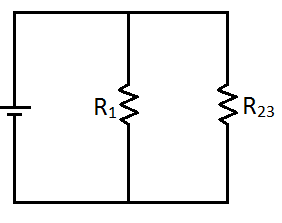Notice that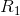and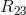are arranged in parallel. To calculate the equivalent resistance of this parallel pair, we use the following equation.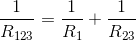Plug in the values, and solve for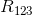.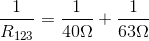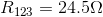This is just like the circuit shown below.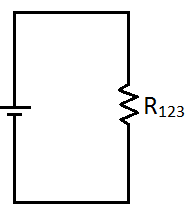### All AP Physics C Electricity Resources